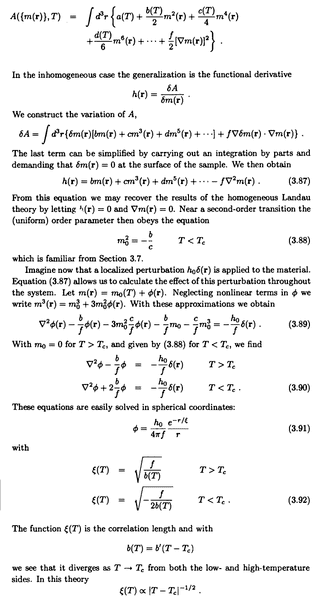# Help understanding textbook on Landau Ginzburg theory

This question is about the Landau Ginzburg of phase transitions which seem to take this classical field theory form.
I don't understand the meaning of the 2nd to last equation
$$b(T) = b'(T -T_c)$$
does that mean b(T) has be redefined in the previous two equations relative to the original definition of ##b(T)##. Does the prime ##\prime## indicate a derivative? Here is the entire case, with ##A## being the free energy of some scalar field m(r), which may be interpreted as magnetization. T is temperature.